Chapter 4, Problem 4.117EP### Organic And Biological Chemistry

7th Edition
STOKER + 1 other
ISBN: 9781305081079

#### Solutions

Chapter
Section### Organic And Biological Chemistry

7th Edition
STOKER + 1 other
ISBN: 9781305081079
Textbook Problem

# Draw structural formulas for the following compounds. a. Thioformaldehyde b. Methanethial c. Thioacetone d. Propanethione

(a)

Interpretation Introduction

Interpretation:

Structural formula of thioformaldeyde has to be drawn.

Concept Introduction:

Carbonyl groups are the one which contain a double bond between carbon and oxygen atom.  Aldehydes and ketones possess this carbonyl functional group in it.  The structural representation of a carbonyl group can be given as shown below,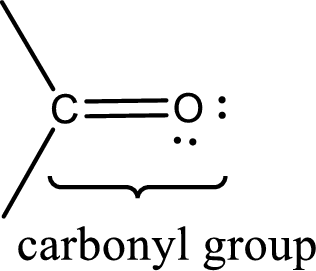If either of the carbon atom or the oxygen atom present in the carbonyl group is substituted by sulfur atom means it is known as sulfur-containing carbonyl compounds.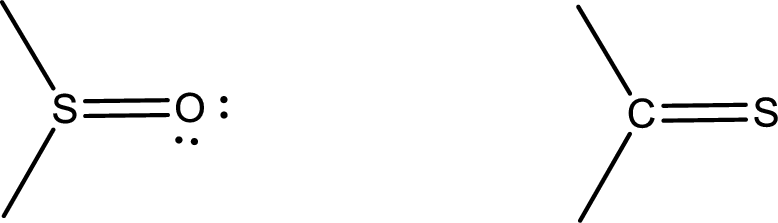Explanation

In a carbonyl compound, the carbon atom or the oxygen atom of the carbonyl group can be replaced by sulfur atom to obtain sulfur containing carbonyl compounds.

If the carbon atom present in the carbonyl group is replaced sulfoxides are obtained.  They are stable compounds.

If the oxygen atom present in the carbonyl group is replaced by sulfur atom, then thiocarbonyl is obtained.  They are thioaldehydes, thioketones.  Thiocarbonyl compounds are less stable and they tend to decompose readily.

Given compound name is thioformaldehyde.  The structural formula of this can be drawn by considering the structure of formaldehyde and replacing the oxygen atom in the carbonyl group by a sulfur atom...

(b)

Interpretation Introduction

Interpretation:

Structural formula of methanethial has to be drawn.

Concept Introduction:

Carbonyl groups are the one which contain a double bond between carbon and oxygen atom.  Aldehydes and ketones possess this carbonyl functional group in it.  The structural representation of a carbonyl group can be given as shown below,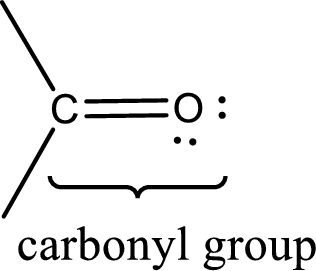If either of the carbon atom or the oxygen atom present in the carbonyl group is substituted by sulfur atom means it is known as sulfur-containing carbonyl compounds.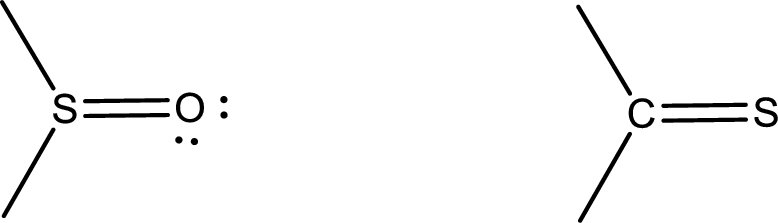(c)

Interpretation Introduction

Interpretation:

Structural formula of thioacetone has to be drawn.

Concept Introduction:

Carbonyl groups are the one which contain a double bond between carbon and oxygen atom.  Aldehydes and ketones possess this carbonyl functional group in it.  The structural representation of a carbonyl group can be given as shown below,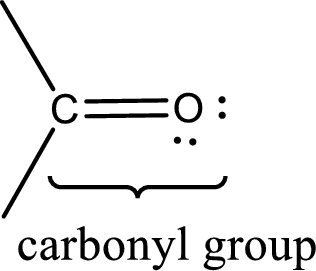If either of the carbon atom or the oxygen atom present in the carbonyl group is substituted by sulfur atom means it is known as sulfur-containing carbonyl compounds.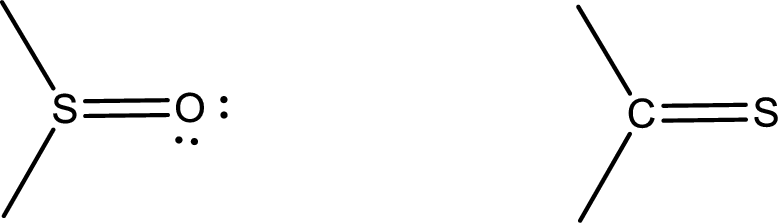(d)

Interpretation Introduction

Interpretation:

Structural formula of propanethione has to be drawn.

Concept Introduction:

Carbonyl groups are the one which contain a double bond between carbon and oxygen atom.  Aldehydes and ketones possess this carbonyl functional group in it.  The structural representation of a carbonyl group can be given as shown below,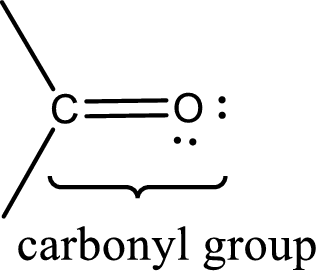If either of the carbon atom or the oxygen atom present in the carbonyl group is substituted by sulfur atom means it is known as sulfur-containing carbonyl compounds.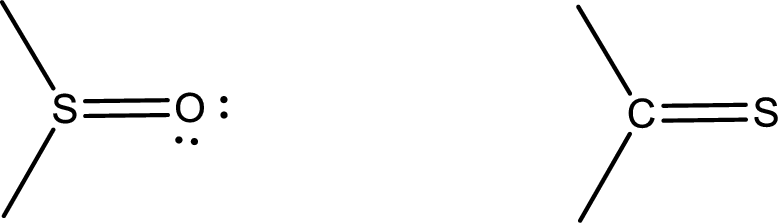### Still sussing out bartleby?

Check out a sample textbook solution.

See a sample solution

#### The Solution to Your Study Problems

Bartleby provides explanations to thousands of textbook problems written by our experts, many with advanced degrees!

Get Started